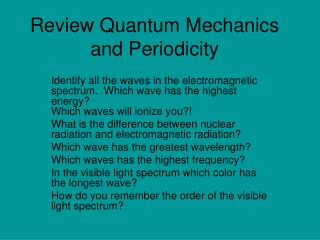# Review Quantum Mechanics and Periodicity - PowerPoint PPT PresentationDownload PresentationReview Quantum Mechanics and Periodicity

Review Quantum Mechanics and PeriodicityDownload Presentation## Review Quantum Mechanics and Periodicity

- - - - - - - - - - - - - - - - - - - - - - - - - - - E N D - - - - - - - - - - - - - - - - - - - - - - - - - - -
##### Presentation Transcript

1. Review Quantum Mechanics and Periodicity Identify all the waves in the electromagnetic spectrum. Which wave has the highest energy? Which waves will ionize you?! What is the difference between nuclear radiation and electromagnetic radiation? Which wave has the greatest wavelength? Which waves has the highest frequency? In the visible light spectrum which color has the longest wave? How do you remember the order of the visible light spectrum?

2. Quantum Mechanics and the PHET Simulation • In the PHET lab what were the colors representing? • What did it mean when the electron traveled up the chart? • What did it mean when an electron dropped down? • How do these motions illustrate the four quantum numbers?

3. Quantum Mechanics and electronice configuration/orbital diagrams Consider the electronic configuration of lead? How many valence electrons does it have? What energy level is the last electron placed in? What sublevel is the last electron placed in? How many orbitals does this sublevel contain? What spin will this electron have? How many sublevels are in each energy level? How many p electrons does Pb have as a neutral atom? Arrange the electronic configuration from nearest orbital to the orbital furthest from the nucleus. What period are the 5d electrons located in? What is the shape of one of the orbitals of a 5d orbital? How many electrons are in the d orbital? How does the orbital diagram of Pb illustrate the four quantum numbers? How does the orbital diagram follow Aufbau ordering, the Pauli Exclusion Principal, Hunds Rule, and the Heisenberg Uncertainty Principal? What period and Block is Pb located in? What other elements have the same reactivity as Pb? Why?

4. Types of Matter • Consider magnesium sulfate (Epsom Salt) dissolved in water How many atoms are in MgSO4? Identify the Epsom Salt as an element or compound? Is it homogeneous or heterogenous? When the Epsom Salt is put in the water is it homogeneous or heterogeneous? Is the bond for magnesium sulfate ionic, covalent, or metallic? If you heated the Epsom salt an a gas evolved leaving a white/brown powder behind, do you think this is a chemical or physical change? Why? Count the protons, electrons, and neutrons for Magnesium. What physical properties would you expect from magnesium. When you heat magnesium and turn it into a liquid(melt it) is this a chemical or physical change? What sub atomic particle identifies magnesium as an element. Draw the Lewis Dot (electron dot) diagram, Bohr diagram (Bulls eye), and electronic configuration for Mg.

5. Quantum Mechanics and electronice configuration/orbital diagrams Consider the electronic configuration of lead. How many valence electrons does it have? 4 What energy level is the last electron placed in? 6 What sublevel is the last electron placed in? p How many orbitals does this sublevel contain? 3 What spin will this electron have? +1/2 How many sublevels are in each energy level? 1 for s, 3 for p, 5 for d, 7 for f. How many p electrons does Pb have as a neutral atom? 26 Arrange the electronic configuration from nearest orbital to the orbital furthest from the nucleus. What period are the 5d electrons located in? 6 What is the shape of one of the orbitals of a 5d orbital? Four leaf clover How many electrons are in all the orientations of the d orbital? 10 How does the orbital diagram of Pb illustrate the four quantum numbers? How does the orbital diagram follow Aufbau ordering, the Pauli Exclusion Principal, Hunds Rule, and the Heisenberg Uncertainty Principal? What period and group is Pb located in? 6 Period, Group 14 What other elements have the same reactivity as Pb? Why? Sn Ge . . Same group